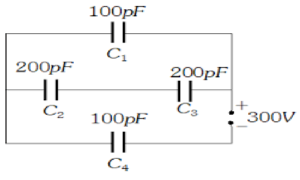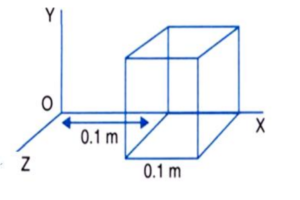# Class 12 Physics Unit Test Sample Paper

Class 12 Physics Unit Test Sample Paper

PHYSICS
CLASS – XII
Total marks:-20

General Instruction: All questions are compulsory.
1. Name the physical quantity whose SI unit is J/C. Is it a scalar or a vector quantity? (1)
2. Define relative permittivity and write its unit also. (1)
3. If the radius of Gaussian surface enclosing a charge is doubled, how does the electric flux through the Gaussian surface change? (1)
4. Write the characteristics of equipotential surface. Draw the equipotential surface due to two positive charges. (2)
5. Determine resultant capacitance of the circuit and charge across each capacitor (2)6. (a) Determine the electrostatic potential energy of a system consisting of two charges 7 μC and –2 μC (and with no external field) placed at (–9 cm, 0, 0) and (9 cm, 0, 0) respectively. (1)
(b) Suppose that the same system of charges is now placed in an external electric field, ( ⁄) ; . What would electrostatic energy of the configuration be?

7. Derive an expression for electric field intensity due to an infinity straight uniformly charged wire. (3)
8. Derive an expression for capacitance of a parallel plate capacitor having dielectric slab inside it. (3)
9. (a) Define electric flux. Write its SI unit. (1)
(b) If electric flux entering and leaving an enclosed surface are and respectively. Determine electric charge inside it (1)
(c) The electric field components due to a charge inside the cube of side 0.1 m are shown: , where , , Calculate (i) the flux through the cube and (ii) charge inside the cube (1)Revised CBSE Syllabus 2021 for Class 12 Physics